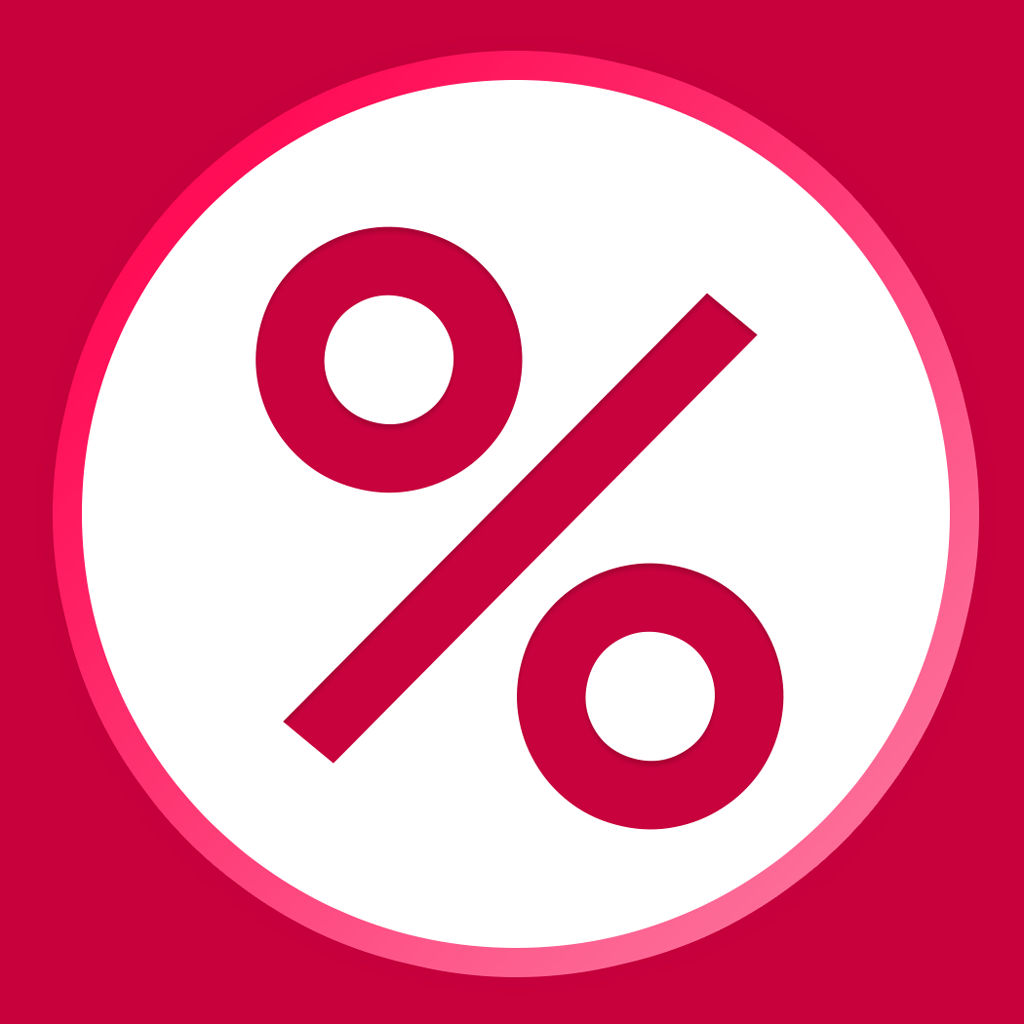Percentage Calculator Free
Removed from the App Store# Percentage Calculator Free

## Thomas Richardson

Free
0

0 Ratings

Update Date

2016-10-13

Size

17.8 MB

### Screenshots for iPhone

Percentage Calculator Free Description
Do you need to know a percentage? Percentage Calculator Free can help.

Our latest application is a powerful tool allowing you to work out a percentage on any number. This can be useful at restaurants, in shops, online and even on general occasions where you need to work out a percentage - fast.

This powerful tool is great for everybody, whether you are good at mathematics, or not. Are you buying an item with a discount? Do you need to work out a tip in a restaurant? We understood this, so we brought a powerful yet easy to use tool to your pocket. Instantly work out the percentage of a number, the percentage of a partial number, the change from one value to another, a number that is a percentage of another value and more - it’s powerful, advanced and free. Have you found a great deal but do not have the time to work the percentage out? Simply open the Percentage Calculator on your phone and have the number that you are looking for within seconds - it’s as easy as 1, 2, 3.

With 8 tools, this makes the free Percentage Calculator application one of the most diverse percentage applications available, and with such powerful features - it’s no wonder that so many trust the Percentage Calculator to help them in everyday life. Tools include:

- The ability to find the percentage of a number:
This simple tool give you the ability to find the percentage of any number - most commonly used when working out the VAT of a product or tip required in a restaurant.

- The ability to find the percentage of a part value:
Another tool that can be used to calculate tips, this tool gives you the ability to work out the percentage of a partial value. For example: what is the percentage of: 50 of 150? Answer: 33.33%.

- The ability to find the percentage change from one value to another:
For example: if an item originally cost 10.00 and now costs 10.99, by what percentage has the value changed?

- The ability to calculate the value that Y% is of value X:
For example: 10 is 20% of which value? Answer: 50

- The ability to increase a number by a percentage:
For example: what is 100 + 69%? Answer: 169

- The ability to decrease a number by a percentage:
For example: what is 100 – 69%? Answer: 31

- The ability to calculate the original value after a percentage has been deducted
For example: If you were to buy a discounted item at 49.99 and the original value was reduced by 45% what was the original value? Answer: 90.89

- The ability to.calculate the original value after a percentage was added.
For example: If an item were to cost £40 what would be the original value if VAT @ 20% was excluded? Answer: 41.66

Experience the powerful and free Percentage Calculator today - work out percentage equations within just a few taps.
Percentage Calculator Free 3.1 Update
2016-10-13 Version History
Fixed formula error that arose in previous update that affected both increase and decrease by calculations

aplogies
More
Price:
Free
Version:
3.1
Size:
17.8 MB
Genre: